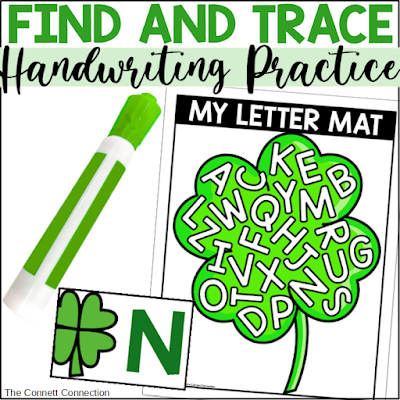## Tuesday, August 9, 2022

### Subtraction Facts Flash Cards and Math Mats for Comparing Differences

Becoming fluent in subtraction is one thing every child will need to move on to higher maths.  Students need to be able to quickly determine the difference.  These math mats give students a variety of ways to practice subtraction fact fluency while also comparing differences of different subtraction problems.

With this subtraction math mat, students will draw a math problem and match it to the difference.  This helps students to work through subtraction problems in a fun way that doesn't involve your typical worksheet.  Students can also see which subtraction problems have the same differences.

With this mat, students will match subtraction problems with the same difference.  They will begin to recognize related subtraction facts using this sorting mat.

This mat allows students to compare the differences between two subtraction problems.  Students will choose two subtraction facts and place them on the mat to make the number sentence true.

Here is another mat for practicing related subtraction mats.  Students will choose two numbers and place them on each side of the mat, then look for subtraction facts with those differences.

MORE WAYS TO CONNECT